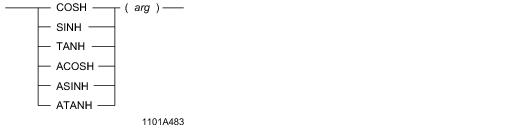# 15.00 - Hyperbolic Functions(COSH, SINH, TANH, ACOSH, ASINH, ATANH) - Teradata Database

## Teradata Database SQL Functions, Operators, Expressions, and Predicates

Product
Release Number
15.00
Content Type
Programming Reference
Publication ID
B035-1145-015K
Language
English (United States)

## Hyperbolic Functions(COSH, SINH, TANH, ACOSH, ASINH, ATANH)

### Purpose

Performs the hyperbolic or inverse hyperbolic function of an argument.

### Syntaxwhere:

 Syntax element … Specifies … arg any real number.

### ANSI Compliance

This is a Teradata extension to the ANSI SQL:2011 standard.

### Result Type and Attributes

Here are the default attributes for the result of hyperbolic and inverse hyperbolic functions.

 Data Type Format Title FLOAT Default format for FLOAT Hyperbolic Cos(arg)Hyperbolic Sin(arg)Hyperbolic Tan(arg)Hyperbolic ArcCos(arg)Hyperbolic ArcSin(arg)Hyperbolic ArcTan(arg)

For information on default data type formats, see SQL Data Types and Literals.

### Result Value

 Function Result COSH(arg) Hyperbolic cosine of arg. SINH(arg) Hyperbolic sine of arg. TANH(arg) Hyperbolic tangent of arg. ACOSH(arg) Inverse hyperbolic cosine of arg. The inverse hyperbolic cosine is the value whose hyperbolic cosine is a number so that: `   acosh(cosh(arg)) = arg` ASINH(arg) Inverse hyperbolic sine of arg. The inverse hyperbolic sine is the value whose hyperbolic sine is a number so that: `   asinh(sinh(arg)) = arg` ATANH(arg) Inverse hyperbolic tangent of arg. The inverse hyperbolic tangent is the value whose hyperbolic tangent is a number so that: `   atanh(tanh(arg)) = arg`

### Argument Types and Rules

If arg is not FLOAT, it is converted to a FLOAT value, based on implicit type conversion rules. If the argument cannot be converted, an error is reported. For more information on implicit type conversion, see “Implicit Type Conversions” on page 583.

If arg is a UDT, the following rules apply:

• The UDT must have an implicit cast to any of the following predefined types:
• Numeric
• Character
• DATE
• To define an implicit cast for a UDT, use the CREATE CAST statement and specify the AS ASSIGNMENT clause. For more information on CREATE CAST, see SQL Data Definition Language.

• Implicit type conversion of UDTs for system operators and functions, including hyperbolic and inverse hyperbolic functions, is a Teradata extension to the ANSI SQL standard. To disable this extension, set the DisableUDTImplCastForSysFuncOp field of the DBS Control Record to TRUE. For details, see Utilities: Volume 1 (A-K).
• Hyperbolic and inverse hyperbolic functions cannot be applied to the following types of arguments:

• BYTE or VARBYTE
• BLOB or CLOB
• CHARACTER or VARCHAR if the server character set is GRAPHIC
• ### Examples

The following are representative hyperbolic and inverse hyperbolic function expressions and results.

 Expression Result COSH(EXP(1)) 7.61012513866229E 000 SINH(1) 1.17520119364380E 000 TANH(0) 0.00000000000000E 000 ACOSH(3) 1.76274717403909E 000 ASINH(LOG(0.1)) -8.81373587019543E -001 ATANH(LN(0.5)) -8.53988047997524E -001